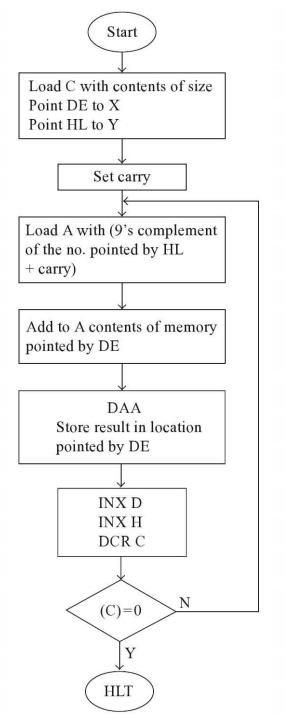Program for subtraction of multi-byte BCD numbers in 8085 Microprocessor

Here we will see one program that can perform subtraction for multi-byte BCD numbers using 8085 microprocessor.

Problem Statement

Write an 8085 Assembly language program to subtract two multi-byte BCD numbers.

Discussion

The numbers are stored into memory, and one additional information is stored. It will show us the byte count of the multi-byte BCD number. Here we are choosing 3-byte BCD numbers. They are stored at location 8001H to 8003H, and another number is stored at location 8004H to 8006H. The location 8000H is holding the byte count. In this case the byte count is 03H.

For the subtraction we are using the 10’s complement method for subtraction.

In this case the numbers are: 672173 – 275188 = 376985

Data

8000
03
8001
73
8002
21
8003
67
8004
88
8005
51
8006
27

Flow DiagramProgram

HEX Codes
Labels
Mnemonics
F000
21, 00, 80

LXI H,8000H
Point to get the count
F003
4E

MOV C,M
Get the count to C
F004
11, 01, 80

LXI D,8001H
Point to first number
F007
21, 04, 80

LXI H,8004H
Point to second number
F00A
37

STC
Set the carry flag
F00B
3E, 99
LOOP
MVI A,99H
Load 99H into A
F00D
CE,00

ACI 00H
Add 00H and Carry with A
F00F
96

SUB M
Subtract M from A
F010
EB

XCHG
Exchange DE and HL
F011
86

Add M to A
F012
27

DAA
F013
77

MOV M,A
Store A to memory
F014
EB

XCHG
Exchange DE and HL
F015
23

INX H
Point to next location by HL
F016
13

INX D
Point to next location by DE
F017
0D

DCR C
Decrease C by 1
F018
C2, 0B, F0

JNZ LOOP
Jump to LOOP if Z = 0
F01B
76

HLT
Terminate the program